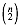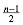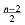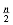Hans Walser, [20090720a]

Dissection of a convex polygon with central symmetry

# 1        General case

A polygon with central symmetry has an even number of vertices.

Theorem:

A convex polygon with central symmetry and 2n vertices can be dissected intoparallelograms.Dissection of a 12-gon

Proof by induction according to the colors [Walser 1983].

We can easily find the dissection by moving copies of the polygon such that every vertex comes once to a particular vertex of the original polygon.First two stepsDissection

# 2        Special cases

## 2.1      Equilateral polygons

An equilateral polygon with central symmetry can be dissected into rhombi.Rhombi

## 2.2      Regular polygons

We discuss now regular polygons with 2n vertices. The dissection we can obtain by moving like above or by rotating the 2n-gon by angles of multiples ofaround a particular vertex ([Lindgren 1972], p. 109, and cover).Dissection of a regular 14-gon

If we are just interested in the vertices of the rhombi, we can work with circles.Circles will work

### 2.2.1    n odd

In this case we getsets, each consisting of n congruent rhombi. The acute angles of the rhombi are.n = 7

### 2.2.2    n even

Solution of M. N. Deshpande’s Problem 1650 in Mathematics Magazine (2002), p. 227 ([Fischer 2003], with beautiful examples).

We getsets, each consisting of n congruent rhombi, and a set ofsquares.n = 6

References

[Lindgren 1972]         Lindgren, H.: Geometric Dissections. Revised and enlarged by Greg Frederickson. New York: Dover 1972.

[Walser 1983]            Walser, Hans: Ein Zerlegungssatz für punktsymmetrische konvexe Vielecke. Elemente der Mathematik (38), 1983, p. 159-160.

Links (2009 / 7 / 20)

[Fischer 2003]